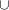### UNION Aggregation Expression

UNION { index-set } set-expression

The UNION aggregation expression evaluates the set-expression for each member of the index set index-set. The result is the set union of the values that are returned by the set-expression. If the index set is empty, then the result is an empty set.

The following statements demonstrate a UNION aggregation. The output is the value of {1,2,3,4}{2,3,4,5}{3,4,5,6}.

```proc optmodel;
put (union{i in 1..3} i..i+3); /* outputs {1,2,3,4,5,6} */
```